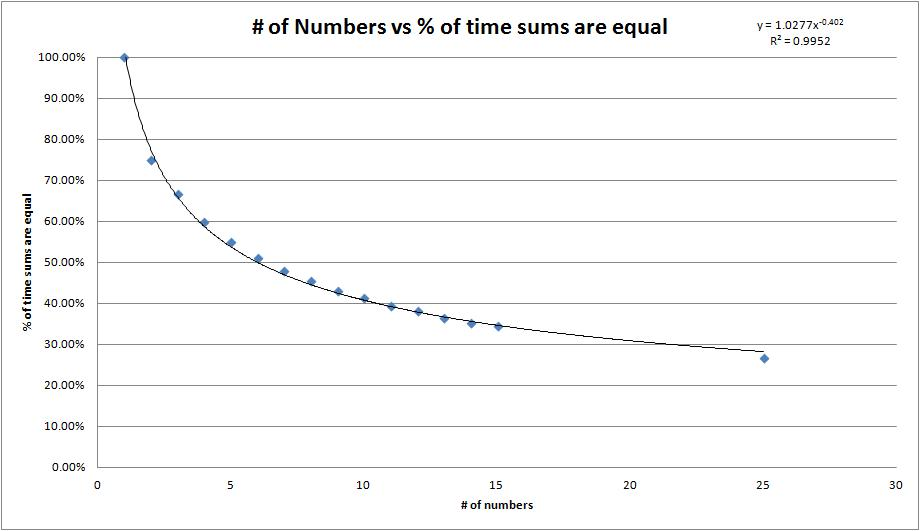If you find any of this useful, please consider donating via PayPal to help keep this site going.# Sum of Rounded vs Rounded Sum

3/10/16

I always wondered about the following question, so I did a simulation to get data and see what I could discover. The question is this, how often can we expect the sum of rounded numbers to equal the rounded sum of the same numbers? This situation can occur in practice. One example, is in a survey publication, where, for a variety of reasons, sums of data in categories may not be equal to the grand total over those categories.

To illustrate, say we have the numbers 1.23, 1.6, and 1.4. These numbers rounded to the nearest integer are 1, 2, and 1, and the sum of these is 4. The original numbers summed up are 1.23 + 1.6 + 1.4 = 4.23, which rounds to 4. So in this example, the sum of the rounded numbers is the same as the rounded sum. However, this is not always the case. For example, use the numbers 1.25, 1.25, and 1.1, and you end up with 3 vs. 4.

I created a spreadsheet to simulate this using Excel and Visual Basic. Check it out here. You first decide how many numbers you want to sum. The random numbers are of the form rand()*10, where rand() is a pseudo-random number between 0 and 1. Next, enter in the number of iterations you want to run the simulation for. Last, simply run the Simulate macro.

Here is a graph showing results from using 1 (trivial) to 15 numbers being summed, as well as 25. I ran 50,000 iterations for each one:Next, I wanted to see what happened to the percent of the time the sum of rounded numbers is equal to the rounded sum by using a larger number of numbers being summed. Here is a table of the results:

 # of numbers in sum % of time sum of rounded numbers and rounded sum are equal 1 100 2 75.02 3 66.63 4 59.90 5 54.97 6 51.13 7 47.99 8 45.51 9 43.05 10 41.34 11 39.40 12 38.22 13 36.44 14 35.17 15 34.43 25 26.66 50 19.12 100 13.86 250 8.64 500 6.40 1,000 4.26 10,000 1.35 100,000 .41

The relationship between number of numbers being summed and the percent of the time the sum of rounded numbers and the rounded sum are equal looks something like f(x) = C*x-p. Whatever the relationship, the percent certainly gets small for any reasonable large number of numbers being summed.

If you enjoyed any of my content, please consider supporting it in a variety of ways: